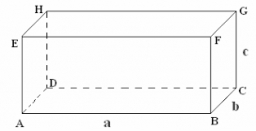# Calculate 19543

Calculate the surface of a prism with a square base whose mantle is a rectangle with sides of 18cm and 8cm. How many solutions does the task have? List all solutions.

S1 =  184.5 cm2
S2 =  152 cm2

### Step-by-step explanation:Did you find an error or inaccuracy? Feel free to write us. Thank you!

Tips for related online calculators
Do you have a linear equation or system of equations and looking for its solution? Or do you have a quadratic equation?

#### Grade of the word problem:

We encourage you to watch this tutorial video on this math problem: